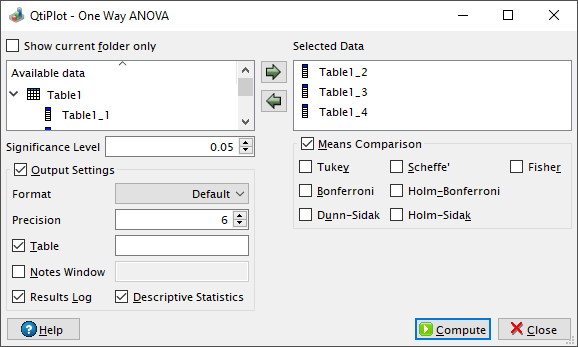One-way ANOVA Dialog

This dialog is activated by selecting the One-Way ANOVA... command from the Statistics -> ANOVA -> menu. The one-way analysis of variance (abbreviated one-way ANOVA) can be used to compare the means of two or more samples using the F distribution. Please visit the Wikipedia article on the one-way ANOVA for more details about this statistical test.

Figure 5-88. The one-way ANOVA dialog.Post-hoc tests

If the controls in the Means Comparison group box are checked QtiPlot can also perform several post-hoc analysis tests. These tests can determine which sample means are significantly different from each other. The folowing post-hoc tests are currently implemented: Tukey, Bonferroni, Dunn-Sidak, Fisher's Least Significant Difference (LSD), Scheffe, Holm-Bonferroni and Holm-Sidak.

The post-hoc tests compare all possible pairs of means, meaning that for N samples there are k = N(N-1)/2 pairs of means to be compared.

For each pairwise comparison QtiPlot computes the difference between the means mi and mj and the Standard Error of the Mean, SEMij = [MSE*(1/ni + 1/nj)]1/2, where MSE is the Mean Square for the Error reported in the ANOVA results table and ni and nj are the sizes of samples i and j respectively. QtiPlot also computes a test statistic based on the difference between the means and on the SEMij, a probability p and a confidence interval (LCL stands for Lower Confidence Limit and UCL stands for Upper Confidence Limit).

The compaired means are said to be significantly different (reported Significance is 1 in the results log/tables) at significance level α (0 ≤ α ≤ 1) if the calculated probability is lower than the value of α chosen by the user.

For each test QtiPlot calculates the test statistics and the probabilities using the following formulas:

1. Tukey:

This test uses the Studentized range statistic q = 21/2|mi - mj|/SEMij.

The probability is calculated using the formula p = 1 - srangecdf(q, DoF, N), where the QtiPlot function srangecdf computes the probability associated with the lower tail of the distribution of the Studentized range statistic for N samples and DoF degrees of freedom (reported in the Error line of the ANOVA results table).

The implementation of the srangecdf function provided by QtiPlot uses code adapted from the following paper by WILLIAM P. DUNLAP, RICHARD S. POWELL and TED K. KONNERTH: A FORTRAN IV function for calculating probabilities associated with the studentized range statistic, published in Behavior Research Methods and Instrumentation, 1977, Vol. 9 (4), 373-375.

2. Bonferroni:

This test uses the statistic t = (mi - mj)/SEMij.

The probability is calculated using the formula p = N(N-1)tcdf(t, DoF), where the tcdf function calculates the lower tail of the cumulative distribution function for the Student's t-distribution with DoF degrees of freedom.

3. Dunn-Sidak:

This test uses the statistic t = (mi - mj)/SEMij.

The probability is calculated using the formula p = 1 - [1 - tcdf(t, DoF)]N(N-1), where the tcdf function calculates the lower tail of the cumulative distribution function for the Student's t-distribution with DoF degrees of freedom.

4. Fisher LSD:

This test uses the statistic t = (mi - mj)/SEMij.

The probability is calculated using the formulas p = 2tcdf(t, DoF) if tcdf(t, DoF) < 0.5 and p = 2[1 - tcdf(t, DoF)] otherwise, where the tcdf function calculates the lower tail of the cumulative distribution function for the Student's t-distribution with DoF degrees of freedom.

5. Scheffe:

This test uses the statistic F = t2, where t = (N-1)1/2(mi - mj)/SEMij.

The probability is calculated using the formula p = 1 - fcdf(F, N-1, DoF), where the fcdf function calculates the lower tail of the cumulative distribution function for the F-distribution with N-1 andDoF degrees of freedom.

6. Holm-Bonferroni:

This test uses the statistic t = (mi - mj)/SEMij.

The probability is calculated using the formulas p = 2tcdf(t, DoF) if tcdf(t, DoF) < 0.5 and p = 2[1 - tcdf(t, DoF)] otherwise, where the tcdf function calculates the lower tail of the cumulative distribution function for the Student's t-distribution with DoF degrees of freedom.

The Holm-Bonferroni test sorts the p-values in ascending order and compares them to nominal significance levels from α/k to α, namely the values: α/k, α/(k-1),...,α/2, α.

7. Holm-Sidak:

This test uses the statistic t = (mi - mj)/SEMij.

The probability is calculated using the formulas p = 2tcdf(t, DoF) if tcdf(t, DoF) < 0.5 and p = 2[1 - tcdf(t, DoF)] otherwise, where the tcdf function calculates the lower tail of the cumulative distribution function for the Student's t-distribution with DoF degrees of freedom.

The Holm-Sidak test sorts the p-values in ascending order and compares them to nominal significance levels from 1 - (1 - α)1/k to α, namely the values: 1 - (1 - α)1/k, 1 - (1 - α)1/(k-1),...,1 - (1 - α)1.